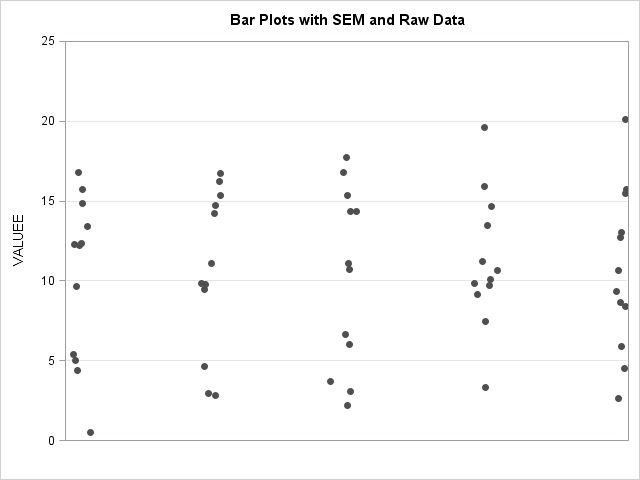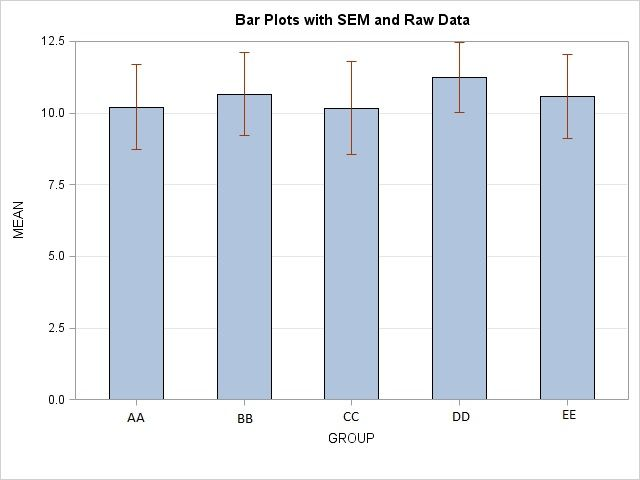## How to combine several SCATTERPLOTs with BARCHARTPARM in PROC TEMPLATE?

I try to plot raw data points and mean bars with standard errors in one graph:

SET long_;

if GROUP = "AA" then AA = -0.955 + 0.05 * rannor(0);

if GROUP = "BB" then BB = 0.25 + 0.05 * rannor(0);

if GROUP = "CC" then CC = 1.5 + 0.05 * rannor(0);

if GROUP = "DD" then DD = 2.75 + 0.05 * rannor(0);

if GROUP = "EE" then EE = 4 + 0.05 * rannor(0);

RUN;

PROC SUMMARY DATA = long NWAY;

CLASS my_class;

VAR my_values;

OUTPUT OUT = summary1 MEAN = MEAN STDERR = STDERR;

RUN;

PROC TEMPLATE;

DEFINE STATGRAPH BarsWithSEMAndRawData;

BEGINGRAPH;

LAYOUT OVERLAY /

YAXISOPTS = (GRIDDISPLAY = ON DISPLAY = (LABEL TICKS TICKVALUES)

LINEAROPTS = (VIEWMIN = 0 THRESHOLDMAX = 1 ) OFFSETMIN = 0 OFFSETMAX = 0)

X2AXISOPTS = (DISPLAY = (LINE) LINEAROPTS = (VIEWMIN = -1 VIEWMAX = 4));

SCATTERPLOT X = AA Y = VALUEE / XAXIS = x2;

SCATTERPLOT X = BB Y = VALUEE / XAXIS = x2;

SCATTERPLOT X = CC Y = VALUEE / XAXIS = x2;

SCATTERPLOT X = DD Y = VALUEE / XAXIS = x2;

SCATTERPLOT X = EE Y = VALUEE / XAXIS = x2;

BARCHARTPARM X = GROUP Y = MEAN / BARWIDTH = 0.5

ERRORLOWER = EVAL(MEAN - STDERR)

ERRORUPPER = EVAL(MEAN + STDERR)

FILLATTRS = (COLOR = LightSteelBlue TRANSPARENCY = 0);

ENDLAYOUT;

ENDGRAPH;

END;

RUN;

When I use the following string:

PROC SGRENDER DATA = long_added TEMPLATE = 'BarsWithSEMAndRawData'; RUN;

only scatters (without labels, ticks, tickvalues) are produced (points.jpg) along with the following message in the SAS Log window:

"WARNING: The BARCHARTPARM statement will not be drawn because one

or more of the required arguments were not supplied.".

When I use the following code:

PROC SGRENDER DATA = summary1 TEMPLATE = 'BarsWithSEMAndRawData'; RUN;

only mean bars and SEM are drawn (bars.jpg) and along with the following message (repeated 5 times) in the SAS Log window:

"WARNING: The SCATTERPLOT statement will not be drawn because one or

more of the required arguments were not supplied.".

How to display scatters and bars with SEM simultaneously in a single graph?

Thank you.4 REPLIES 4Jay54
Meteorite | Level 14

## Re: How to combine several SCATTERPLOTs with BARCHARTPARM in PROC TEMPLATE?

As you see, the SGRENDER procedure takes only one data set.  So, all the data columns needed for the barchartparm statement and all the scatterplot statements must be in one data set.  If the data sets have unique column names, a simple merge of the two data sets may work.  Jittering is supported in SAS 9.3.

## Re: How to combine several SCATTERPLOTs with BARCHARTPARM in PROC TEMPLATE?

Happy New YearIndeed, the data sets have unique variable names. A common name I used to combine the data sets by different approaches:

DATA my_data_combined;

MERGE data_set_1 data_set_2;

BY GROUP;

RUN;

DATA my_data_combined;

SET data_set_1 data_set_2;

BY GROUP;

RUN;

DATA my_data_combined;

SET data_set_1 data_set_2;

RUN;

But the same message in SAS Log window appeared the same:

WARNING: The data for a BARCHARTPARM statement are not appropriate.

The BARCHARTPARM statement expects summarized data. The bar

chart might not be drawn correctly.

I used the following piece of code for that statement:

BARCHARTPARM

X = GROUP

Y = MEAN /

ERRORLOWER = EVAL(MEAN - STDERR)

ERRORUPPER = EVAL(MEAN + STDERR);

The my_data_combined data set ( created with both SET and BY ) is in the attached file.

What it can be?Jay54
Meteorite | Level 14

## Re: How to combine several SCATTERPLOTs with BARCHARTPARM in PROC TEMPLATE?

This is a different message from previous.  Previously, the BarChartParm and ScatterPlot were not drawn because some required variables were not provided.  Now, the BarChartParm is saying you have extra observations per category.

## Re: How to combine several SCATTERPLOTs with BARCHARTPARM in PROC TEMPLATE?

If I'm not mistaken your comment is reasonable in the case of SET and SET-BY approaches when indeed there is one extra observation per category. But when I use MERGE-BY statements the message is the same. I.e. there are no extra records, but they are multiplied according to the number of observations in a category...

Discussion stats
• 4 replies
• 1853 views
• 0 likes
• 2 in conversation